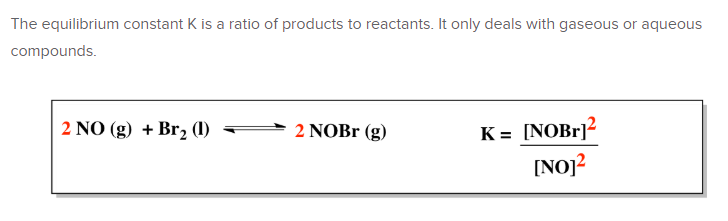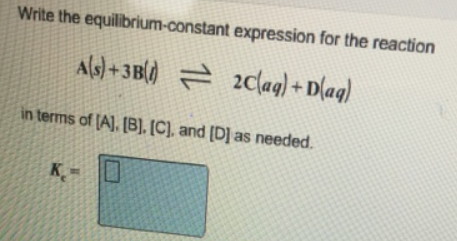# Problem: Write the equilibrium-constant expression for the reaction A (s) + 3B (l) ⇌ 2C (aq) + D (aq)in terms of [A], [B], [C), and [D] as needed.

###### FREE Expert Solution94% (416 ratings)###### Problem Details

Write the equilibrium-constant expression for the reaction

A (s) + 3B (l) ⇌ 2C (aq) + D (aq)

in terms of [A], [B], [C), and [D] as needed.